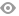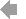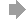Basic HTML VersionView Full Version
Page 219 - Course_Catalog_2020-2021
P. 219

All courses are for unit credit and apply to a De Anza associate degree unless otherwise noted.
5 Units
Partial derivatives, multiple integrals, vector calculus. As an honors course the students will be expected to complete extra assignments to gain deeper insight in calculus.
MATH 1DH Calculus - HONORS
This course is an introduction to data analysis making use of graphical and numerical techniques to study patterns and departures from patterns. The student studies randomness with an emphasis on understanding variation, collects information in the face of uncertainty, checks distributional assumptions, tests hypotheses, uses probability as a tool for anticipating what the distribution of data may look like under a set of assumptions, and uses appropriate statistical models to draw conclusions from data. The course introduces the student to applications in engineering, business, economics, medicine, education, social sciences, psychology, the sciences, and those pertaining to issues of contemporary interest. The use of technology (computers or graphing calculators) will be required in certain applications. Where appropriate, the contributions to the development of statistics by men and women from diverse cultures will be introduced. MATH 10 and therefore Math 10H is a required lower-division course for students majoring or minoring in many disciplines such as data science, nursing, business, and others. Because this is an honors course, the students will be expected to complete extra assignments to gain deeper insight into probability and statistics.
MATH 11 Finite Mathematics 5 Units
(See general education pages for the requirement this course meets.) Prerequisite: Intermediate Algebra (MATH 109, MATH 114 or MATH 130)
or equivalent.
Five hours lecture (60 hours total per quarter).
Application of linear equations, sets, matrices, linear programming, mathematics of finance and probability to real-life problems. Emphasis on the understanding of the modeling process, and how mathematics is used in real-world applications.
MATH 11H Finite Mathematics - HONORS 5 Units
(See general education pages for the requirement this course meets.)
(Not open to students with credit in MATH 11.)
(Admission into this course requires consent of the Honors Program Coordinator.) Prerequisite: Intermediate Algebra (MATH 109, MATH 114 or MATH 130)
or equivalent.
Five hours lecture (60 hours total per quarter).
Application of linear equations, sets, matrices, linear programming, mathematics of finance and probability to real-life problems. Emphasis on the understanding of the modeling process, and how mathematics is used in real-world applications. Because this is an honors course the students will be expected to complete extra assignments to gain deeper insight in Finite Mathematics.
MATH 12 Introductory Calculus for Business 5 Units and Social Science
(See general education pages for the requirement this course meets.) Prerequisite: MATH 11, 11H, 31, 31B, 41 or 41H.
Five hours lecture (60 hours total per quarter).
Introduction to limits, differentiation, and integration of single variable functions. Differentiation of multivariate functions. Applications in business, economics, and social science.
MATH 17 Integrated Statistics 2 5 Units
(Formerly MATH 57.)
(See general education pages for the requirement this course meets.) Prerequisite: MATH 217.
Five hours lecture (60 hours total per quarter).
This is the second quarter of two in the Statway sequence comprised of MATH 217 and MATH 17. This sequence covers concepts and methods of statistics with an emphasis on data analysis. Sequence topics include methods for collecting data, graphical and numerical descriptive statistics, correlation, simple linear regression, basic concepts of probability, probability distributions, confidence intervals, hypothesis tests for means and proportions, chi-square tests, and ANOVA. The course introduces students to applications in engineering, business, economics, medicine, education, the sciences, and those pertaining to issues of contemporary interest. Where appropriate, the contributions to the development of statistics by men and women from diverse cultures will be introduced. This sequence is recommended for students with majors that require no mathematics beyond freshman-level statistics. It is not appropriate for students with majors in math, science, computer science or business, nor for students desiring to attend private universities.
MATH 22 Discrete Mathematics 5 Units
(See general education pages for the requirement this course meets.) Prerequisite: MATH 32, 43 or 43H with a grade of C or better or equivalent, and CIS 22A or CIS 35A with a grade of C or better or equivalent.
Five hours lecture (60 hours total per quarter).
Elements of discrete mathematics with applications to computer science. Topics include methods of proof, mathematical induction, logic, sets, relations, graphs, combinatorics, and Boolean algebra.
M (See general education pages for the requirement this course meets.)
(Not open to students with credit in MATH 1D.)
(Admission into this course requires consent of the Honors Program Coordinator.) Prerequisite: MATH 1C or MATH 1CH (with a grade of C or better) or equivalent. Advisory: EWRT 211 and READ 211, or ESL 272 and 273.
Five hours lecture (60 hours total per quarter).
MATH 2A Differential Equations
(See general education pages for the requirement this course meets.) (Not open to students with credit in MATH 2AH.)
Prerequisite: MATH 1D or MATH 1DH (with a grade of C or better). Advisory: EWRT 211 and READ 211, or ESL 272 and 273.
Five hours lecture (60 hours total per quarter).
Ordinary differential equations and selected applications.
MATH 2AH Differential Equations - HONORS
5 Units
5 Units
(See general education pages for the requirement this course meets.)
(Not open to students with credit in MATH 2A.)
(Admission into this course requires consent of the Honors Program Coordinator.) Prerequisite: MATH 1D or MATH 1DH (with a grade of C or better).
Five hours lecture (60 hours total per quarter).
Ordinary differential equations and selected applications. As an honors course the students will be expected to complete extra assignments to gain deeper insight in differential equations.
MATH 2B Linear Algebra
(See general education pages for the requirement this course meets.) (Not open to students with credit in MATH 2BH.)
Prerequisite: MATH 1D or MATH 1DH (with a grade of C or better). Advisory: EWRT 211 and READ 211, or ESL 272 and 273.
Five hours lecture (60 hours total per quarter).
Linear algebra and selected topics of mathematical analysis.
MATH 2BH Linear Algebra - HONORS
5 Units
5 Units
(See general education pages for the requirement this course meets.)
(Not open to students with credit in MATH 2B.)
(Admission into this course requires consent of the Honors Program Coordinator.) Prerequisite: MATH 1D or MATH 1DH (with a grade of C or better).
Five hours lecture (60 hours total per quarter).
Linear algebra and selected topics of mathematical analysis. As an honors course the students will be expected to complete extra assignments to gain deeper insight into linear algebra.
MATH 10 Introductory Statistics 5 Units
(See general education pages for the requirement this course meets.) (Not open to students with credit in MATH 10H.)
Prerequisite: Intermediate Algebra (MATH 109, MATH 114 or MATH 130) or equivalent.
Five hours lecture (60 hours total per quarter).
This course is an introduction to data analysis making use of graphical and numerical techniques to study patterns and departures from patterns. The student studies randomness with an emphasis on understanding variation, collects information in the face of uncertainty, checks distributional assumptions, tests hypotheses, uses probability as a tool for anticipating what the distribution of data may look like under a set of assumptions, and uses appropriate statistical models to draw conclusions from data. The course introduces the student to applications in engineering, business, economics, medicine, education, social sciences, psychology, the sciences, and those pertaining to issues of contemporary interest. The use of technology (computers or graphing calculators) will be required in certain applications. Where appropriate, the contributions to the development of statistics by men and women from diverse cultures will be introduced. This Statistics course is a required lower- division course for students majoring or minoring in many disciplines such as data science, nursing, business, and others.
MATH 10H Introductory Statistics - HONORS 5 Units
(See general education pages for the requirement this course meets.)
(Not open to students with credit in MATH 10.)
(Admission into this course requires consent of the Honors Program Coordinator.) Prerequisite: Intermediate Algebra (MATH 109, MATH 114 or MATH 130)
or equivalent.
Advisory: EWRT 211 and READ 211, or ESL 272 and 273. Five hours lecture (60 hours total per quarter).
218
2O20-2O21 DE ANZA COLLEGE CATALOG217   218   219   220   221